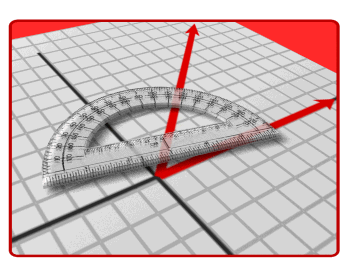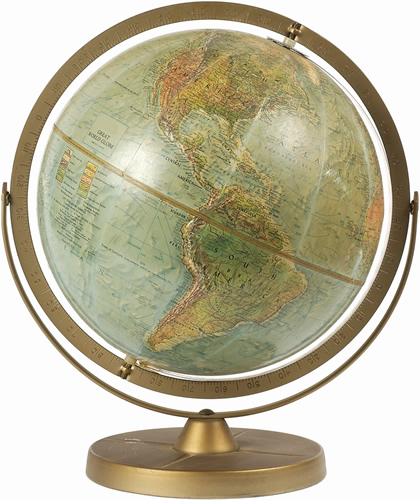•

##Units for this year are:

Place value of whole and decimal numbers

Addition, subtraction, multiplication, and division of whole numbers and decimals

Classifying 3D figures

Volume

Fractions as division; addition/subtraction of fractions with like and unlike denominators; multiplication and division of fractions

Problem solving with whole numbers and decimals

Representing data with fractions

Problem solving with fractions

Algebraic expressions

Equations and inequalities

Rate, ratios and proportionality

Statistics and distribution

Customary and metric measurementSocial Studies